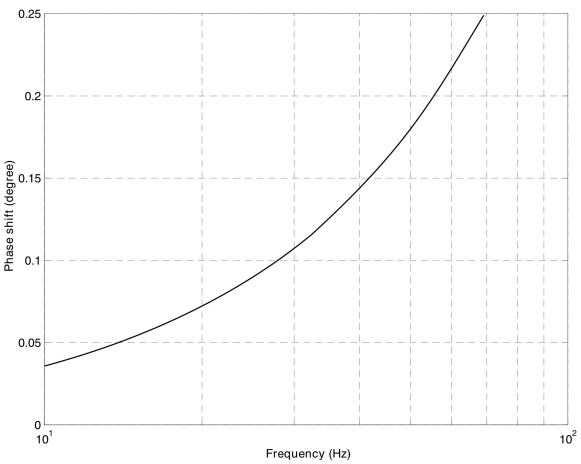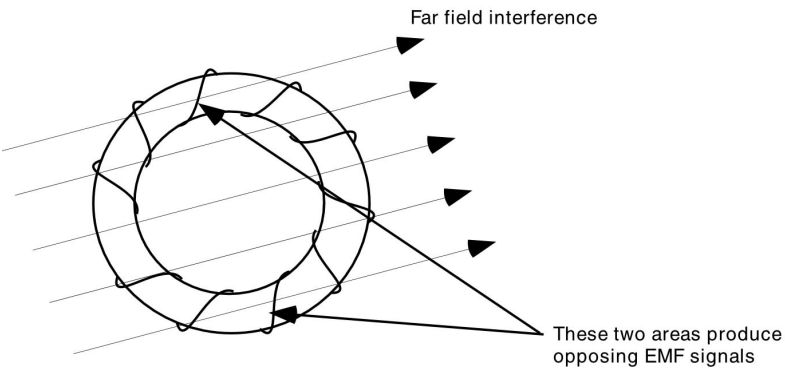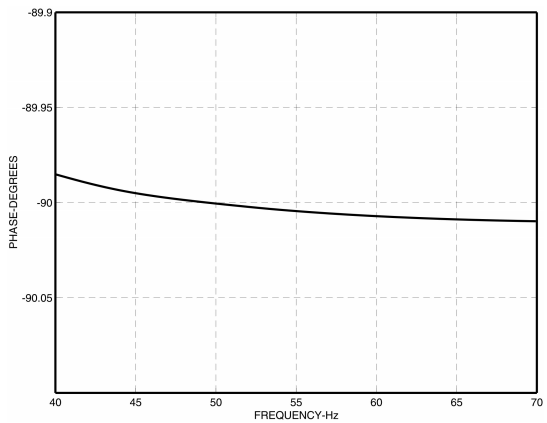# Current Sensing for Energy Metering

Solid-state electric energy meters contain both voltage and current sensing elements. The current sensing requirement is a more difficult problem. Not only does the current sensor require a wider measurement dynamic range, it also needs to handle a wider frequency range because of the rich harmonic contents in the current waveform.

This paper shows how a digital integrator can be used to convert the di/dt signal output from Rogowski coil current sensor to an appropriate signal and how it can be combined for a high-current energy meter.

### Introduction

Today, most advanced solid-state energy meters adopt mixed-signal architecture, using high accuracy A/D converter front end and DSP back-end. Some implementations use discrete components while the majority uses ASIC designed specifically for energy measurement. This mixed-signal architecture offers superb accuracy and long-term stability. Before voltage and current are sampled, both signals need to be conditioned to the appropriate signal level. All energy meters contain both voltage and current sensing elements. Current sensing is a more difficult problem. Current sensor requires wider measurement dynamic range, it also needs to handle a much wider frequency range because of the rich harmonic contents in the current waveform. As the energy consumption in a household continue to increase, the needs for measuring high current is no longer limited to industrial applications. For example, the new energy meters installed in the U.S. residential market need to measure maximum current up to 200A. Today’s current sensing technologies can no longer measure current as high as this very cost-effectively.

Rogowski coil has long been used for high current measurement such as in sub-station transformers and arc wielding machines. It offers numerous advantages comparing with the other current sensing solutions. However, the difficulty for building an analog integrator which is stable over a long period of time has kept Rogowski coil from being used in metering applications. This article introduces the basic principle of a Rogowski coil and a recent digital implementation of an integrator. This combination has enabled this current sensing technology to be successfully used in a recent high-current electric energy meter design. Because of the many advantages to this technology, this could be the sensor of choice for the next generation electric energy meters.

### Today’s current sensing solutions

The three most common sensor technologies today are the low resistance current shunt, the current transformer (CT), and the Hall effect sensor.

#### Low Resistance Current Shunt

Current shunt is the lowest cost solution available today. A simple model for this current measurement device is shown in Figure 1.Figure 1. A simple model of the shunt with parasitic inductance

Low resistance current shunt offers good accuracy at low cost and the current measurement is simple. When performing high precision current measurement, one must consider the parasitic inductance of the shunt. The inductance is typically in the order of only a few nH. It affects the shunt’s impedance magnitude at relatively high frequency. However, its effect on phase is significant enough, even at line frequency, to cause noticeable error at low power factor. Figure 2 shows the phase shift resulting from a 2nH inductance in a 200µΩ shunt.Figure 2. Phase shift caused by the self-inductance of a shunt (2nH in a 200µΩ shunt)

The percentage measurement error caused by any phase mismatch between the voltage and current signal paths can be approximated by the following formula:

In the above expression, φ represents the power factor phase angle between the voltage and current. As one can see, a phase mismatch of 0.1° will result in about 0.3% error at power factor of 0.5. Therefore, special care needs to be taken to ensure phase are precisely matched between the internal signal paths for the voltage and current.

Shunt is rather low cost and reliable. It is a popular choice for energy metering applications. However, because the current shunt is fundamentally a resistive element, the heat it generates is proportional to the square of current passing through. This self-heating problem makes shunt a rarity among high current energy meters.

#### Current Transformer (CT)

Current Transformer (CT) is a transformer which converts the primary current into a smaller secondary current. CT is the most common sensor among today’s high current solid-state energy meters. CT can measure up to very high current and consumes little power. Because of the magnetizing current, CT typically have a small phase shift associated with it (0.1°-0.3°). If un-calibrated, it will lead to noticeable error at low power factor (see earlier discussion on parasitic inductance in current shunt). In addition, the ferrite material used in the core can saturate at high current. Once magnetized, the core will contain hysteresis and the accuracy will degrade unless it is demagnetized again. Figure 3 show a typical hysteresis curve of a ferrite material.

CT saturation can occur when current surges beyond a CT’s rated current, or when there is substantial dc component in the current (e.g. when driving a large half-wave
rectified load). Today’s solution to the saturation problem is to use ferrite material with very high permeability. This typically involves using Mu-metal core. However, this type of CT’s have inconsistent and larger phase shift comparing with the conventional iron core CT’s. Energy meters based on Mu-metal core CT’s would require multiple calibration points for both current level and temperature variations.

#### Hall Effect Sensor

There are two main types of Hall effect sensors: open-loop and closed-loop implementation. Most Hall effect sensors found in energy meters use open-loop design for lower system cost. Hall effect sensor has outstanding frequency response and is capable of measuring very large current. However, the drawbacks of this technology include that the output from Hall effect sensor has a large temperature drift and it usually requires an stable external current source. Hall effect sensors are somewhat less common comparing with the CT.

### Rogowski coil

A simple Rogowski coil is an inductor which has mutual inductance with the conductor carrying the primary current. Rogowski coil is typically made from aircore coil so in theory there is no hysteresis, saturation, or non-linearity.

If current i(t) passes through a long straight wire on z-axis, the magnetic field at a random point P which has coordinate (ρ, θ, z) in cylindrical coordinate is:

The electromotive force (EMF) generated by the magnetic field in any area in space can be calculated using Maxwell’s equation:

Figure 4 shows an example of Rogowski coil current sensor. It consists of N-turns of rectangular air-core coil arranged around a long straight wire and perpendicular to the magnetic field generated by the current in the wire.

The EMF of the coil in this arrangement is:

The constant term M represents the mutual inductance of the Rogowski coil, and it has a unit of Henry (H). It indicates the signal level from the output of the coil per unit di/dt. The voltage output of the coil relies only on the di/dt changes in primary current. Because EMF is only generated when there is changes in the magnetic field, Rogowski coil cannot be used to measure dc component in the current. In addition, this type of sensor can easily measure ac current up to thousands of Amps. That is why it is so useful in many high current measuring applications. It has no iron core so there is no non-linearity over a very wide measurement range (from hundreds of amps to milliamps).

The basic operating principle of a Rogowski coil is to measure the primary current through mutual inductance. Because Rogowski coil relies on measuring magnetic field, it makes this type of current sensor susceptible to external magnetic field interference comparing with the CT. The following highlights a few important aspects to minimize external magnetic field interference.

#### Minimizing unwanted loop area

Any loop formed by conductor will pick up magnetic field. It is therefore important to minimize unwanted loop area to reduce interference pick-up. For example, Figure 5 shows a toroidal air-core Rogowski coil. It is intended to detect the magnetic field around the circular ring. However, the winding itself makes up an undesirable loop and makes this design susceptible to interference perpendicular to the ring.

#### Design with interference cancellation

Interference is generally far field in nature and therefore will be more uniformly distributed throughout the sensor. It is important for the Rogowski coil to differentiate between the far field interference and the near field signal, and design the coil in such a way that the far field interference will cancel out within the coil. For example, the circular shape of the toroidal coil ensures that there is opposing EMF when a far field interference is applied to the coil.Figure 6. Far field interference generate opposing EMF in different part of the coil

However, note that perfect cancellation will require a perfectly uniform winding and
zero impedance of the coil. In practice, the small non-uniformity of the winding and
non-zero coil wire impedance will create some interference susceptibility in the
Rogowski coil.

#### Shielding

Shielding can be used to add extra protection. However, to shield magnetic field of frequency as low as the power line frequency requires thick shielding or high permeability in the shielding material to be used. If care is taken when designing the Rogowski coil, shielding can be avoided.

### Designing the Integrator

#### The analog approach

Because the output from the Rogowski coil is proportional to the time derivative of the current, an integrator is needed to convert the di/dt signal back to the format of i(t) for further processing. Traditional approach has been to use high performance op-amps and build an analog integrator. Figure 7 shows a simple integrator design using an op-amp.

The biggest challenge of this analog implementation has been to design an integrator that is accurate over the long operating life and the hostile operating environment of a meter. This was one of the major drawback which had prevented Rogowski coil from being widely adopted, even among the traditional high current industrial meters.

#### Digital Integrator

To overcome this problem. A digital implementation has recently been introduced. In frequency domain, an integration can be viewed as a -20dB/decade attenuation and a constant –90° phase shift. A digital implementation can implement this with outstanding accuracy. Figures 8 and 9 are the frequency response and the detailed phase response of the digital integrator implemented in Analog Devices’ ADE7759 energy measurement ASIC.Figure 8. Magnitude response of a digital integrator from 10Hz to 10kHz (with gain normalized to 0dB at 60Hz)Figure 9. Phase response of a digital integrator (from 40Hz to 70Hz)

As shown, the phase and magnitude response of a digital integrator is very close to ideal. When interfacing with an IC which has on-chip digital integrator, building a meter with the Rogowski coil is just as simple as using current sensors such as CT or shunt. The air-core coil has no hysteresis, saturation, or non-linearity problem. In addition, it has outstanding ability to handle large current. The added benefit of the digital implementation is that it is more stable over time and environmental changes. These are very important for energy metering application because of the hostile operating condition and long operation life of an energy meter. A residential energy meter with maximum current of 200Amps that is based on the Rogowski coil and ADE7759 has recently been introduced. Extensive in-house experiments has shown that this new meter design outperforms meters that are based on traditional current sensing technologies in many areas.

Figure 10 below shows a linearity accuracy plot of the ADE7759 with a Rogowski coil current sensor over a 1000:1 (60dB) dynamic range. It has less than 0.1% over this wide dynamic range.